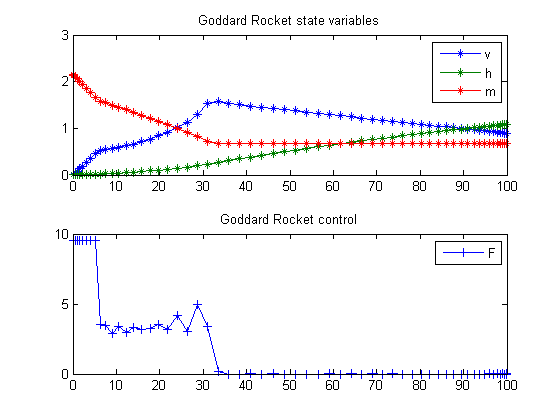45  Goddard Rocket, Maximum Ascent, Final time fixed, Singular solution

Example 7.2 (ii) from the paper: H. Maurer, "Numerical solution of singular control problems using multiple shooting techniques", Journal of Optimization Theory and Applications, Vol. 18, No. 2, 1976, pp. 235-257

Remark: You can vary the fixed final time t_f to obtain Fig. 8 in the paper

L.G. van Willigenburg, W.L. de Koning

Copyright (c) 2007-2009 by Tomlab Optimization Inc.

45.1  Problem setup

toms t

% Parameters
aalpha = 0.01227; bbeta = 0.145e-3; c = 2060; g0 = 9.81;
r0 = 6.371e6; r02=r0*r0; m0 = 214.839; mf = 67.9833; Fm = 9.525515;
t_f = 100; %Paper value 206.661;

45.2  Solve the problem, using a successively larger number of collocation points

nvec = [20 40 60];
for i=1:length(nvec);
n = nvec(i);

p = tomPhase('p', t, 0, t_f, n);
setPhase(p);
tomStates h v m
tomControls F

% Initial guess
if i==1
x0 = {icollocate({v == 10*t; h == 10*t^2
m == m0+(t/t_f)*(mf-m0)})
collocate(F == Fm)};
else
x0 = {icollocate({v == vopt; h == hopt
m == mopt})
collocate(F == Fopt)};
end

% Box constraints
cbox = {icollocate({0 <= v; 0 <= h
mf <= m <= m0
0 <= F <= Fm})};

% Boundary constraints
cbnd = {initial({v == 0; h == 0; m == m0})
final({m == mf})};

D = aalpha*v.^2.*exp(-bbeta*h);
g = g0; % or g0*r02./(r0+h).^2;

% ODEs and path constraints
ceq = collocate({dot(h) == v
m*dot(v) == F*c-D-g*m
dot(m) == -F});

% Objective
objective = -final(h);

45.3  Solve the problem

options = struct;
options.name = 'Goddard Rocket';
if i==1
options.solver = 'multiMin';
options.xInit = 20;
end
%options.scale = 'auto'
solution = ezsolve(objective, {cbox, cbnd, ceq}, x0, options);

% Optimal v and more to use as starting guess
vopt = subs(v, solution);
hopt = subs(h, solution);
mopt = subs(m, solution);
Fopt = subs(F, solution);
Problem type appears to be: lpcon
Starting numeric solver
===== * * * =================================================================== * * *
TOMLAB - Tomlab Optimization Inc. Development license  999001. Valid to 2011-02-05
=====================================================================================
Problem: ---  1: Goddard Rocket - Trial 1       f_k -108076.039985832960000000
sum(|constr|)      0.000067118000448545
f(x_k) + sum(|constr|)-108076.039918714960000000

Solver: multiMin with local solver snopt.  EXIT=4.  INFORM=5.
Find local optima using multistart local search
Nonlinear infeasible problem. TotFuncEv 1. TotConstrEv 209

FuncEv    1 ConstrEv  209 ConJacEv  209 Iter   76
CPU time: 0.421875 sec. Elapsed time: 0.453000 sec.
Warning: Solver returned ExitFlag = 4
The returned solution may be incorrect.
Problem type appears to be: lpcon
Starting numeric solver
===== * * * =================================================================== * * *
TOMLAB - Tomlab Optimization Inc. Development license  999001. Valid to 2011-02-05
=====================================================================================
Problem: ---  1: Goddard Rocket                 f_k -108220.931724708310000000
sum(|constr|)      0.000369534980940259
f(x_k) + sum(|constr|)-108220.931355173320000000
f(x_0)-108076.039985832410000000

Solver: snopt.  EXIT=0.  INFORM=1.
SNOPT 7.2-5 NLP code
Optimality conditions satisfied

FuncEv    1 ConstrEv   20 ConJacEv   20 Iter   19 MinorIter  536
CPU time: 0.234375 sec. Elapsed time: 0.235000 sec.
Problem type appears to be: lpcon
Starting numeric solver
===== * * * =================================================================== * * *
TOMLAB - Tomlab Optimization Inc. Development license  999001. Valid to 2011-02-05
=====================================================================================
Problem: ---  1: Goddard Rocket                 f_k -108245.171256515870000000
sum(|constr|)      0.000090842481984530
f(x_k) + sum(|constr|)-108245.171165673380000000
f(x_0)-108220.931724708060000000

Solver: snopt.  EXIT=0.  INFORM=1.
SNOPT 7.2-5 NLP code
Optimality conditions satisfied

FuncEv    1 ConstrEv   33 ConJacEv   33 Iter   22 MinorIter 1129
CPU time: 0.656250 sec. Elapsed time: 0.672000 sec.
end

t = subs(collocate(t),solution);
v = subs(collocate(vopt),solution);
h = subs(collocate(hopt),solution);
m = subs(collocate(mopt),solution);
F = subs(collocate(Fopt),solution);

45.4  Plot result

subplot(2,1,1)
plot(t,v/1e3,'*-',t,h/1e5,'*-',t,m/1e2,'*-');
legend('v','h','m');
title('Goddard Rocket state variables');

subplot(2,1,2)
plot(t,F,'+-');
legend('F');
title('Goddard Rocket control');